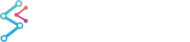﻿ Fibonacci Retracement Drawing Tool | WPF Chart DocumentationSciChart WPF 2D Charts > The Trading Drawing Tools API > Fibonacci Retracement Drawing Tool
Fibonacci Retracement Drawing Tool

FibonacciRetracementAnnotation is an annotation useful for trading charts based on a Fibonacci Ratio.  To create this, you have to draw a TrendLine between two extreme points. Then the vertical distance between these two points will be divided by Fibonacci Ratio levels that are placed at key levels (that corresponds to 0, 23.6, 38.2, 50, 61.8, 76.4 and 100%).

NOTE: The Drawing Tools API in SciChart.Charting.DrawingTools is available in the Enterprise and SDK Editions of SciChart onlyAs the FibonacciRetracementAnnotation is a CompositeAnnotation (annotation that consists of a number of other annotations) it has no special properties, only common properties of usual annotations.

The FibonacciRetracementAnnotation consists of:

• Trendline – which is a LineAnnotation
• FibonacciTable – which is a CompositeAnnotation that consists of multiple of FibonacciRatioLine annotations.
• FibonacciRatioLine has all properties of HorizontalLineAnnotation and one special property, called “LineLevel”. This property corresponds to a level value(in percentage).

## Adding FibonacciRetracementAnnotation via mouse click

To add a FibonacciRetracementAnnotation via mouse click you have to add TradingAnnotationCreationModifier to your SciChartSurface.ChartModifier and set AnnotationType as FibonacciRetracementAnnotation. Please see the article on the TradingAnnotationCreationModifier for how to use this type.

## Declaring a FibonacciRetracementAnnotation in XAML

To declare a FibonacciRetracementAnnotation in XAML you have to declare the annotation and then declare its InitialBasePoints collection that is actually collection of ComparablePoint objects. ComparablePoint stores X and Y values that are used for placing annotation point.

Declaring a FibonnacciRetracementAnnotation in XAML
Copy Code
```<!-- XAxis, YAxis, RenderableSeries omitted for brevity -->
<s:SciChartSurface.Annotations>

<!--Declare FibonacciRetracementAnnotation-->
<s:FibonacciRetracementAnnotation IsEditable="True">

<!-- Declare InitialBasePoints collection that is used for
creating annotation from XAML.
InitialBasePoints is actually observable collection of
ComparablePoint-s. ComparablePoint stores X and Y point Value.
-->
<s:FibonacciRetracementAnnotation.InitialBasePoints>
<!-- Placing first point -->
<s:ComparablePoint X="2"  Y="4"/>
<!-- Placing second point -->
<s:ComparablePoint X="9"  Y="-4"/>

</s:FibonacciRetracementAnnotation.InitialBasePoints>
</s:FibonacciRetracementAnnotation>
</s:SciChartSurface.Annotations>
```

Please Note that the FibonacciRetracementAnnotation has limit of two base points. As you can see here, you just have to set this two points for FibonacciRetracementAnnotation: [X1=2; Y1=4] and [X2=9; Y2=-4].

## Declaring a FibonacciRetracementAnnotation in Code-Behind

To add FibonacciRetracementAnnotation in code, simply use the following C# code:

Declaring a FibonnacciRetracementAnnotation in Code
Copy Code
```// Create a SciChartSurface. XAxis, YAxis omitted for brevity
var sciChartSurface = new SciChartSurface();
// Create a FibonacciRetracementAnnotation.
var fibonacciRetracement = new FibonacciRetracementAnnotation();
// FibonacciRetracementAnnotation should be added to
//SciChartSurface.Annotation collection first, to make SetBasePoint() method
//works correctly
sciChart.Annotations.Add(fibonacciRetracement);
// Set first point of FibonacciRetracementAnnotation
fibonacciRetracement.SetBasePoint(2, 4);
// Set second point of FibonacciRetracementAnnotation
fibonacciRetracement.SetBasePoint(9, -4);
```

## Declaring a FibonacciRetracementAnnotation in MVVM

To add a FibonacciRetracementAnnotation via MVVM please follow the instructions for adding annotations with MVVM: Declaring Annotations in MVVM with the AnnotationsBinding Markup Extension

To add a FibonacciRetracementAnnotation via a ViewModel you have to use SciChart markup extension called AnnotationsBinding,

The AnnotationsBinding Markup Extension
Copy Code
```<s:SciChartSurface Annotations="{s:AnnotationsBinding Annotations}">
</s:SciChartSurface>
```

Now you need to create collection for Annotations in ViewModel. Then create and add a FibonacciRetracementAnnotationViewModel to that collection of Annotations like this:

Declaring a FibonnacciRetracementAnnotation in a ViewModel
Copy Code
```public class MyViewModel : INotifyPropertyChanged
{
public ObservableCollection<IAnnotationViewModel> Annotations { get; }

public void Foo()
{
// Create a FibonacciRetracementAnnotation.
fibonacciRetracement = new FibonacciRetracementAnnotationViewModel();
// FibonacciRetracementAnnotation should be added to
// Annotations collection first, to make SetBasePoint() method
// works correctly
Annotations.Add(fibonacciRetracement);
// Set first point of FibonacciRetracementAnnotation
fibonacciRetracement.SetBasePoint(2, 4);
// Set second point of FibonacciRetracementAnnotation
fibonacciRetracement.SetBasePoint(9, -4);
}
}
```